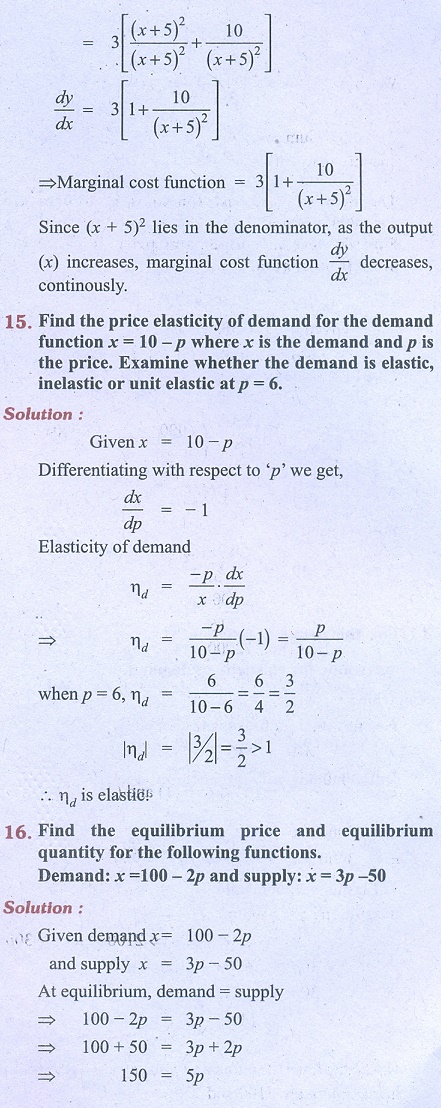Home | | Business Maths 11th std | Exercise 6.1: Demand, supply, cost, revenue and profit functions, Elasticity

# Exercise 6.1: Demand, supply, cost, revenue and profit functions, Elasticity

Business Mathematics and Statistics Book back answers and solution for Exercise questions - Mathematics: Applications of Differentiation: Demand, supply, cost, revenue and profit functions, ElasticityTags : Problem Questions with Answer, Solution | Applications of Differentiation | Mathematics , 11th Business Mathematics and Statistics(EMS) : Chapter 6 : Applications of Differentiation
Study Material, Lecturing Notes, Assignment, Reference, Wiki description explanation, brief detail
11th Business Mathematics and Statistics(EMS) : Chapter 6 : Applications of Differentiation : Exercise 6.1: Demand, supply, cost, revenue and profit functions, Elasticity | Problem Questions with Answer, Solution | Applications of Differentiation | Mathematics